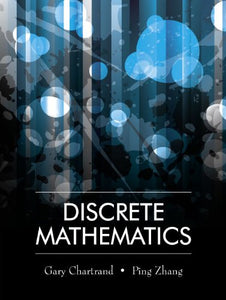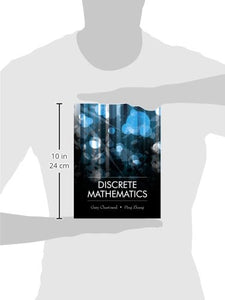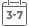# Discrete Mathematics

Vendor
Brand: Waveland Pr Inc
Regular price
\$137.07
Sale price
\$76.71
Quantity must be 1 or more

Attention: For textbook, access codes and supplements are not guaranteed with used items.Chartrand and Zhang's Discrete Mathematics presents a clearly written, student-friendly introduction to discrete mathematics. The authors draw from their background as researchers and educators to offer lucid discussions and descriptions fundamental to the subject of discrete mathematics. Unique among discrete mathematics textbooks for its treatment of proof techniques and graph theory, topics discussed also include logic, relations and functions (especially equivalence relations and objective functions), algorithms and analysis of algorithms, introduction to number theory, combinatorics (counting, the Pascal triangle, and the binomial theorem), discrete probability, partially ordered sets, lattices and Boolean algebras, cryptography, and finite-state machines. This highly versatile text provides mathematical background used in a wide variety of disciplines, including mathematics and mathematics education, computer science, biology, chemistry, engineering, communications, and business.

Some of the major features and strengths of this textbook: Numerous carefully explained examples and applications facilitate learning, More than 1,600 exercises, ranging from elementary to challenging, are included with hints/answers to all odd-numbered exercises, Descriptions of proof techniques are accessible and lively, Students benefit from the historical discussions throughout the textbook, An Instructor's Solutions Manual contains complete solutions to all exercises.

0. What Is Discrete Mathematics?
1. Logic Statements
2. Sets
3. Methods of Proof
4. Mathematical Induction
5. Relations and Functions
6. Algorithms and Complexity
7. Integers
8. Introduction to Counting